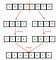# Merge Sort a linked list

Sorting algorithms are frequently asked during a technical interview. Several common sorting algorithms such as quicksort, selection sort, merge sort are usually applied to sorting a list. However, sorting a linked list is not straightforward: it’s not easy to access an element by index in constant running time. Most of the sorting algorithms ask for extra space and the running time is relatively large. Today, I’ll talk about how to apply the merge sort algorithm to a linked list, the running time is O(n*log(n)), and it happens in-place.

## What is Merge Sort?

Merge sort is a divide and conquer algorithm that sorts the input list recursively. There are two steps to finish the process:

• Divide the input list into n sub-list, each sub-list has the same size.
• Merge the sub-lists into one big list, and each element in the list is ordered.

Here’s a picture illustrating the process:merge sort process

As we can see from the picture, there are two key steps to sort a list: 1). divide a list evenly; 2).merge the sub-list in the end. I’ll talk about how to achieve these functions in a linked list.

## How to divide a linked list into two parts?

The most intuitive way is to count the total_size of the linked list, then divide the linked list into two parts, each part has the size of total_size / 2. However, we found it takes O(n) time to run because we must iterate the whole linked list in the beginning. The more efficient way is applying two-pointers approaching. Assume we have two pointers: a fast pointer and a slow pointer. In each step, the fast pointer steps across two nodes, the slow pointer only steps across one node. When the fast node approaches the end of the list, the slow pointer is actually standing at the middle point of the linked list. Let’s prove it together:

## How to merge two linked lists in ascending order?

Assume we have two sorted linked lists, we want to merge them as a big sorted list. We can have two pointers to indicate the node we’re currently comparing, and append the smaller node into the new node. Let’s see how it works:

## Merge sort a linked list

Now it’s time to build the whole solution with previous key functions. Remember it’s a divide and conquer algorithm, recursion may happen in many places!

## Analyze:

• Time Complexity: `O(nlogn)`, where n is the number of nodes in the linked list.
• Space Complexity: `O(nlogn)`, where n is the number of nodes in the linked list. Since the problem is recursive, we need additional space to store the recursive call stack. The maximum depth of the recursion tree is `O(nlogn)` .

## Reference

on the way to become a programmer

## More from Wangyy

on the way to become a programmer